# From Least Squares to k-Nearest Neighbor (kNN)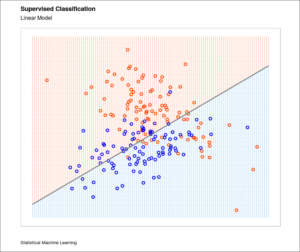The linear model is one of the most widely used data science tools and one of the most important.  In contrast, there is another basic tool:  thenearest neighbor method (NN).  Prediction and classification are two uses for these models.  In practice, classification results (ie. feature classes) are used by machines in many ways: to recognize faces in a crowd, to “read” road signs by distinguishing one letter from another and to set voter registration districts by separating population groups.  This article applies and compares linear and non-linear classification methods

#### Introduction by Comparison

A side-by-side comparison of methods reveals their nature:

• The linear model fit using least squares makes strict assumptions about the structure of the model and the underlying data.  A closed form matrix equation solves linear regression.  The resulting model fit is typically stable, and predications tend to be inflexible in shape and possibly inaccurate;
• Thenearest neighbor method makes only limited assumptions about the model and data.  Iterative search functions solve nearest neighbor problems. The resulting model fit is very flexible in shape, predictions are usually accurate, but prone to instability.

#### Least-Squares Classification

For example, Figure 1 shows a scatter plot of training data on the input pairand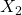, each of which has a 100 points. The random data can be used to define the output class variable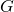, which sets the color of the background grid with the values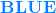and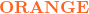.

First, a linear least squares model applied the training data to define the response variable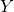and to determine the class of the background grid space.  Secondly, the input values ofare coded as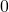fordata points andfordata points. Finally, the fitted values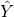define the class variablegiven the rule:

(1)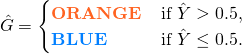Hence, the two-dimensional classification problem in matrix notation is:

(2)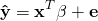with no intercept term and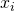equal toordepending on the class. The regression line defines the decision boundary given by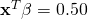. In summary, the background grid is shaded in Figure 1 given the classification defined by ().

Its clear, however, that there are several mis-classifications in the data on both sides of the linear decision boundary in Figure 1. As a result, the decision boundary is too inflexible.  More likely, the optimal decision boundary is non-linear and maybe disjoint in nature.

####-Nearest Neighbor MethodIn contrast, thenearest neighbor method uses the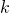observations in the training set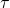closest to the pointon the background space grid to form. TheNN fit foris defined by:

(3)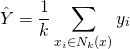where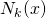is the neighborhood of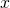with theclosest pointsin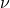. Closeness implies a metric, which for simplicity is Euclidean distance. So, in words, we find theobservations for the feature grid pointclosest toin the input space, and average their responses.

In Figure 2, the training data is the same as that in Figure 1.  However, the feature space is now classified using the average class of the 25-nearest-neighbors given equation (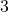) as the method of fitting. It is now obvious that the decision boundary that separates thefrom theregion is far more irregular, and responds to local clusters and outliers where one class dominates.

Asa result, in Figure 2, with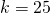, there are far fewer training observations that are misclassified than in Figure 1. This doesn’t give much comfort, though, since the classification boundary is still prone to errors.

in Figure 3, with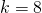, we now see less errors.  The classification boundary also has disjoint islands to help separate the data.

In figure 4, none of the training data are misclassified with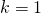. A little thought suggests that for k-nearest-neighbor fits, the error on the training data should be an increasing function of, and will always be 0 for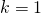.

#### Final Comparison and Takeaways

The-nearest-neighbor fits have a single parameter, the number of neighbors,.  Linear least squares relies on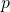, the number of model parameters. The number of parameters for-nearest neighbors is.  This is generally much bigger than, and decreases with increasing. To get an idea of why, note that if the neighborhoods were nonoverlapping, there would beneighborhoods and we would fit one parameter (a mean) in each neighborhood.

Finally, unlike linear least squares, the sum-of-squared errors on the training set has no merit as a criterion for pickingin the nearest neighbor method, since we would always pickas the best answer.  However, the boundary for any solution with a low value is unstable, complex and noisy.

This entry was posted in Data Science, Modeling, R Programming, Website. Bookmark the permalink.